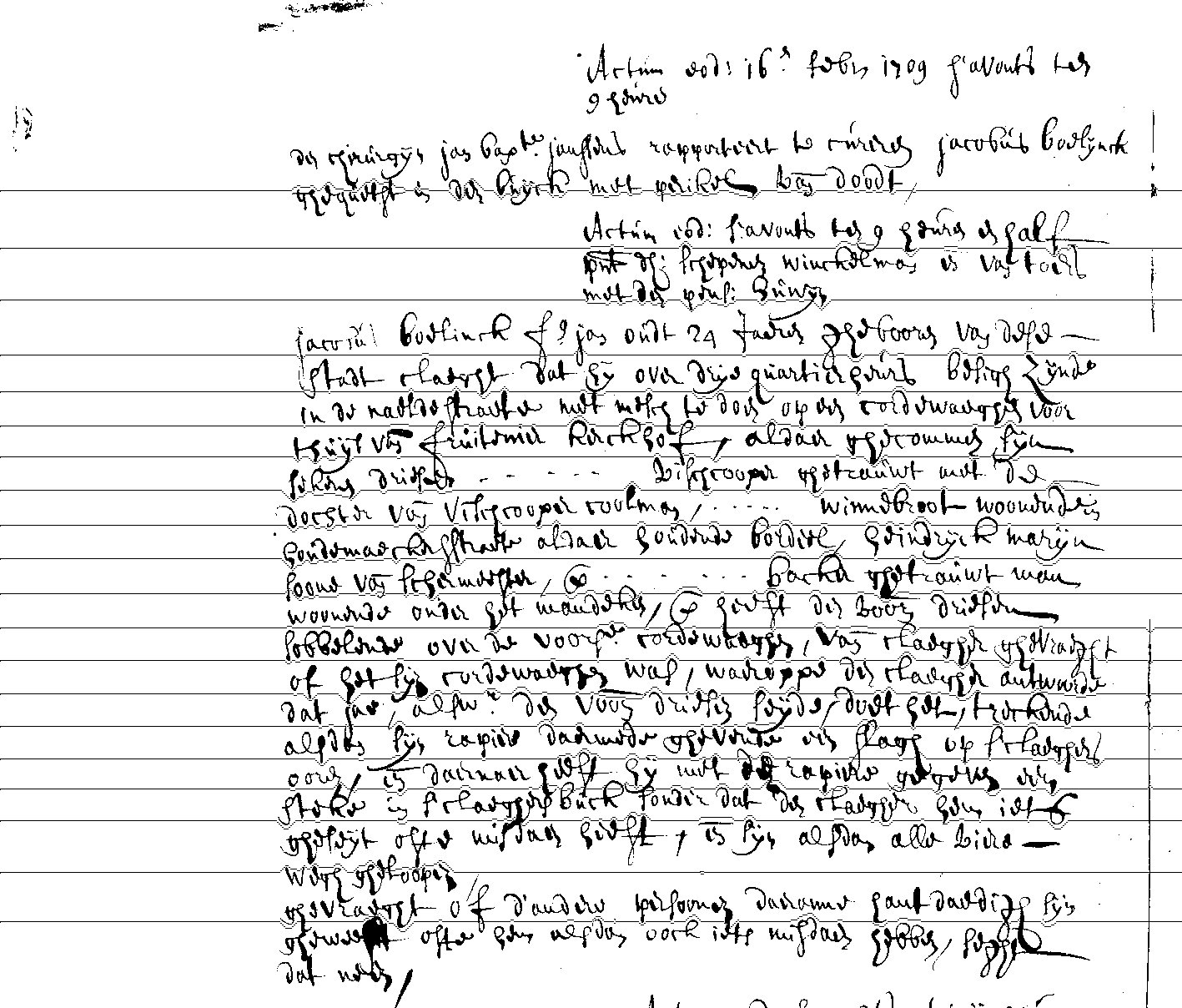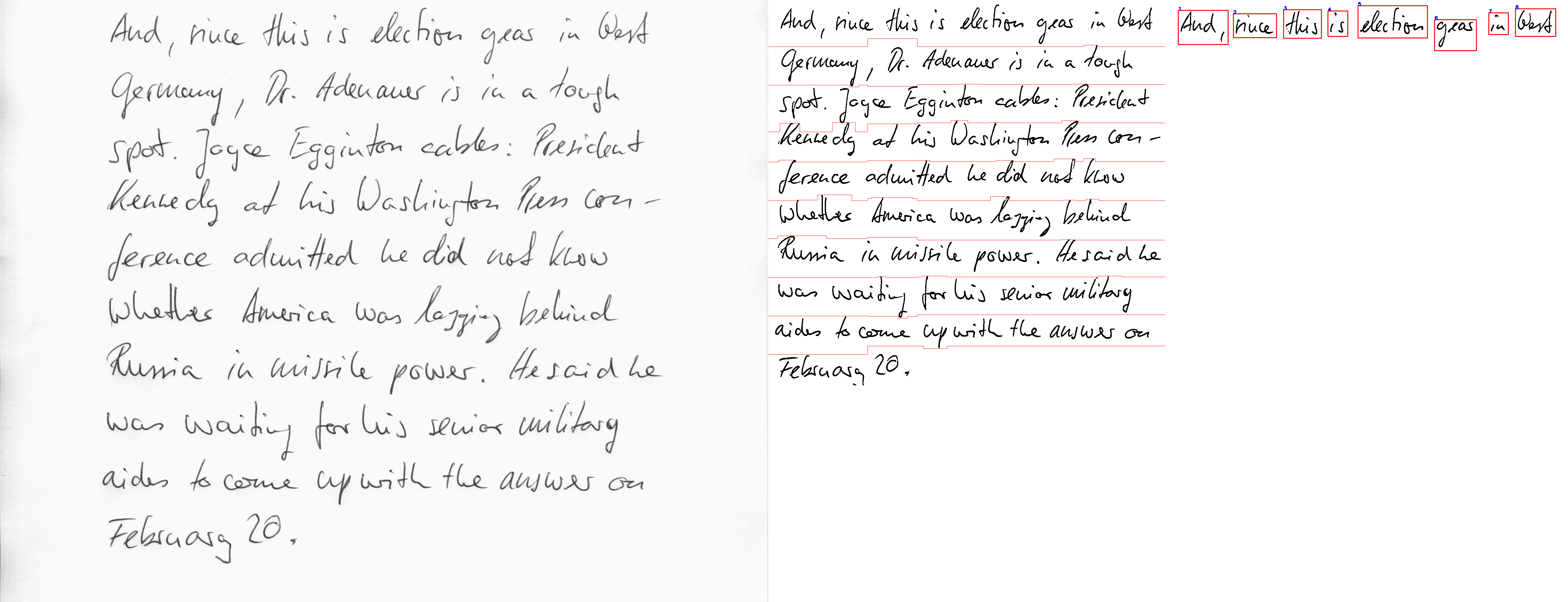# image.textlinedetector

The image.textlinedetector R package detects text lines in digital images and segments these into words.

Objective of the package is to more easily plug the text lines in Handwritten Text Recognition modelling frameworks like the one explained in this document

The algorithm in this R package follows the following techniques:

1. An Implementation of a Novel A* Path Planning Algorithm for Line Segmentation of Handwritten Documents paper link
2. A Statistical approach to line segmentation in handwritten documents paper link

More descriptions of technique 2 can be found in this document

### Installation

• For installing the development version of this package and to execute the example
``````install.packages("opencv")
install.packages("magick")
install.packages("image.binarization")
remotes::install_github("DIGI-VUB/image.textlinedetector")``````

Look to the documentation of the functions

``help(package = "image.textlinedetector")``

## Example

### Based on the paper `An Implementation of a Novel A* Path Planning Algorithm for Line Segmentation of Handwritten Documents```````library(opencv)
library(magick)
library(image.binarization)
library(image.textlinedetector)
#path <- "C:/Users/Jan/Desktop/OCR-HTR/RABrugge_TBO119_693_088.jpg"
path  <- system.file(package = "image.textlinedetector", "extdata", "example.png")
img   <- image_binarization(img, type = "su")
areas <- image_textlines_astar(img, morph = TRUE, step = 2, mfactor = 5)
areas <- lines(areas, img, channels = "bgr")
areas\$n
areas\$overview
areas\$lines
areas\$textlines[]
areas\$textlines[]
combined <- lapply(areas\$textlines, FUN = function(x) image_read(ocv_bitmap(x)))
combined <- do.call(c, combined)
combined``````

### Based on the paper `A Statistical approach to line segmentation in handwritten documents```````library(opencv)
library(magick)
library(image.binarization)
library(image.textlinedetector)
path   <- system.file(package = "image.textlinedetector", "extdata", "example.png")
img
img_bw <- image_binarization(img, type = "isauvola")
areas  <- image_textlines_flor(img, light = TRUE, type = "sauvola")
areas\$overview
areas\$textlines[]
areas  <- lines(areas, img_bw, channels = "gray")
textwords <- image_wordsegmentation(areas\$textlines[])
textwords\$n
textwords\$overview
textwords\$words[]
textwords\$words[]
combined <- lapply(textwords\$words, FUN = function(x) image_read(ocv_bitmap(x)))
combined <- do.call(c, combined)
combined``````

### DIGI

By DIGI: Brussels Platform for Digital Humanities: https://digi.research.vub.be